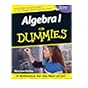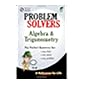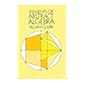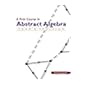Normal view MARC view ISBD view

# The algebra & trigonometry problem solver : a complete solution guide to any textbook / staff of Research and Education Association, M. Fogiel, director ; special chapter reviews by Jerry Shipman.

Material type:BookSeries: REA's problem solvers.Copyright date: Piscataway, N.J. : REA, 2000Edition: First Edition.Description: xiv, 915 pages : illustrations ; 26 cm.ISBN: 0878915087; 9780878915088.Other title: Algebra and trigonometry problem solver | Algebra & trigonometry [Cover title].DDC classification: 512.0076
Partial contents:
1. Fundamental algebraic laws and operations -- 2. Least common multiple / greatest common divisor -- 3. Sets and subsets -- 4. Absolute values -- 5. Operations with fractions -- 6. Base, exponent, power -- 7. Roots and radicals -- 8. Algebraic addition, subtraction, multiplication, division -- 9. Functions and relations -- 10. Solving linear equations -- 11. Properties of straight lines -- 12. Linear inequalities -- 13. Systems of linear equations and inequalities -- 14. Determinants and matrices -- 15. Factoring expressions and functions -- 16. Solving quadratic equations by factoring -- 17. Solutions by quadratic formula -- 18. Solving quadratic inequalities -- 19. Graphing quadratic equations / conics and inequalities -- 20. Systems of quadratic equations -- 21. Equations and inequalities of degree greater than two -- 22. Progressions and sequences -- 23. Mathematical induction -- 24. Factorial notation -- 25. Binomial theorem/expansion -- 26. Logarithms and exponentials -- 27. Trigonometry -- 28. Inverse trigonometric functions -- 29. Trigonometric equations -- 30. Polar coordinates -- 31. Vectors and complex numbers -- 32. Analytic geometry -- 33. Permutations -- 34. Combinations -- 35. Probability -- 36. Series -- 37. Decimal/fractional conversions scientific notation -- 38. Areas and perimeters -- 39. Angles of elevation, depression, and azimuth -- 40. Motion -- 41. Mixtures/fluid flow -- 42. Numbers, digits, coins, and consecutive integers -- 43. Age and work -- 44. Ratio, proportions, and variations -- 45. Costs -- 46. Interest and investments -- 47. Problems in space.
Tags from this library: No tags from this library for this title.
Item type Current location Call number Copy number Status Date due Item holdsLibro académico
512.0076 A394 2000 (Browse shelf) Ej. 1 Available
Total holds: 0
##### Browsing Biblioteca del Campus Shelves Close shelf browser512 S8388a 2001 Algebra I for dummies / 512 S8388a 2001 Algebra I for dummies / 512 S8388a 2001 Algebra I for dummies / 512.0076 A394 2000 The algebra & trigonometry problem solver : 512.02 C5921e 1984 Elements of abstract algebra / 512.02 F812f 2003 A first course in abstract algebra / 512.02 G168c 2017 Contemporary abstract algebra /

Includes index.

Issues for 2007- cataloged as a serial in LC. DLC

1. Fundamental algebraic laws and operations -- 2. Least common multiple / greatest common divisor -- 3. Sets and subsets -- 4. Absolute values -- 5. Operations with fractions -- 6. Base, exponent, power -- 7. Roots and radicals -- 8. Algebraic addition, subtraction, multiplication, division -- 9. Functions and relations -- 10. Solving linear equations -- 11. Properties of straight lines -- 12. Linear inequalities -- 13. Systems of linear equations and inequalities -- 14. Determinants and matrices -- 15. Factoring expressions and functions -- 16. Solving quadratic equations by factoring -- 17. Solutions by quadratic formula -- 18. Solving quadratic inequalities -- 19. Graphing quadratic equations / conics and inequalities -- 20. Systems of quadratic equations -- 21. Equations and inequalities of degree greater than two -- 22. Progressions and sequences -- 23. Mathematical induction -- 24. Factorial notation -- 25. Binomial theorem/expansion -- 26. Logarithms and exponentials -- 27. Trigonometry -- 28. Inverse trigonometric functions -- 29. Trigonometric equations -- 30. Polar coordinates -- 31. Vectors and complex numbers -- 32. Analytic geometry -- 33. Permutations -- 34. Combinations -- 35. Probability -- 36. Series -- 37. Decimal/fractional conversions scientific notation -- 38. Areas and perimeters -- 39. Angles of elevation, depression, and azimuth -- 40. Motion -- 41. Mixtures/fluid flow -- 42. Numbers, digits, coins, and consecutive integers -- 43. Age and work -- 44. Ratio, proportions, and variations -- 45. Costs -- 46. Interest and investments -- 47. Problems in space.

There are no comments for this item.